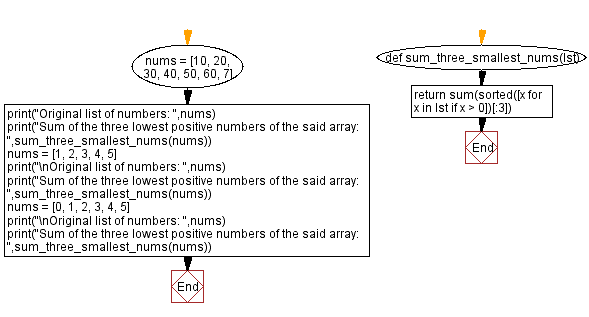﻿ Python: Compute the sum of the three lowest positive numbers from a given list of numbers - w3resource

# Python: Compute the sum of the three lowest positive numbers from a given list of numbers

## Python Basic - 1: Exercise-89 with Solution

Write a Python program to compute the sum of the three lowest positive numbers from a given list of numbers.

Sample Solution:

Python Code:

``````def sum_three_smallest_nums(lst):
return sum(sorted([x for x in lst if x > 0])[:3])
nums = [10, 20, 30, 40, 50, 60, 7]
print("Original list of numbers: ",nums)
print("Sum of the three lowest positive numbers of the said array: ",sum_three_smallest_nums(nums))
nums = [1, 2, 3, 4, 5]
print("\nOriginal list of numbers: ",nums)
print("Sum of the three lowest positive numbers of the said array: ",sum_three_smallest_nums(nums))
nums = [0, 1, 2, 3, 4, 5]
print("\nOriginal list of numbers: ",nums)
print("Sum of the three lowest positive numbers of the said array: ",sum_three_smallest_nums(nums))
``````

Sample Output:

```Original list of numbers:  [10, 20, 30, 40, 50, 60, 7]
Sum of the three lowest positive numbers of the said array:  37

Original list of numbers:  [1, 2, 3, 4, 5]
Sum of the three lowest positive numbers of the said array:  6

Original list of numbers:  [0, 1, 2, 3, 4, 5]
Sum of the three lowest positive numbers of the said array:  6
```

Pictorial Presentation:Flowchart:Python Code Editor:

Have another way to solve this solution? Contribute your code (and comments) through Disqus.

What is the difficulty level of this exercise?

Test your Programming skills with w3resource's quiz.

﻿